# Natural Images Statistics¶

Important: Please read the installation page for details about how to install the toolboxes. $\newcommand{\dotp}{\langle #1, #2 \rangle}$ $\newcommand{\enscond}{\lbrace #1, #2 \rbrace}$ $\newcommand{\pd}{ \frac{ \partial #1}{\partial #2} }$ $\newcommand{\umin}{\underset{#1}{\min}\;}$ $\newcommand{\umax}{\underset{#1}{\max}\;}$ $\newcommand{\umin}{\underset{#1}{\min}\;}$ $\newcommand{\uargmin}{\underset{#1}{argmin}\;}$ $\newcommand{\norm}{\|#1\|}$ $\newcommand{\abs}{\left|#1\right|}$ $\newcommand{\choice}{ \left\{ \begin{array}{l} #1 \end{array} \right. }$ $\newcommand{\pa}{\left(#1\right)}$ $\newcommand{\diag}{{diag}\left( #1 \right)}$ $\newcommand{\qandq}{\quad\text{and}\quad}$ $\newcommand{\qwhereq}{\quad\text{where}\quad}$ $\newcommand{\qifq}{ \quad \text{if} \quad }$ $\newcommand{\qarrq}{ \quad \Longrightarrow \quad }$ $\newcommand{\ZZ}{\mathbb{Z}}$ $\newcommand{\CC}{\mathbb{C}}$ $\newcommand{\RR}{\mathbb{R}}$ $\newcommand{\EE}{\mathbb{E}}$ $\newcommand{\Zz}{\mathcal{Z}}$ $\newcommand{\Ww}{\mathcal{W}}$ $\newcommand{\Vv}{\mathcal{V}}$ $\newcommand{\Nn}{\mathcal{N}}$ $\newcommand{\NN}{\mathcal{N}}$ $\newcommand{\Hh}{\mathcal{H}}$ $\newcommand{\Bb}{\mathcal{B}}$ $\newcommand{\Ee}{\mathcal{E}}$ $\newcommand{\Cc}{\mathcal{C}}$ $\newcommand{\Gg}{\mathcal{G}}$ $\newcommand{\Ss}{\mathcal{S}}$ $\newcommand{\Pp}{\mathcal{P}}$ $\newcommand{\Ff}{\mathcal{F}}$ $\newcommand{\Xx}{\mathcal{X}}$ $\newcommand{\Mm}{\mathcal{M}}$ $\newcommand{\Ii}{\mathcal{I}}$ $\newcommand{\Dd}{\mathcal{D}}$ $\newcommand{\Ll}{\mathcal{L}}$ $\newcommand{\Tt}{\mathcal{T}}$ $\newcommand{\si}{\sigma}$ $\newcommand{\al}{\alpha}$ $\newcommand{\la}{\lambda}$ $\newcommand{\ga}{\gamma}$ $\newcommand{\Ga}{\Gamma}$ $\newcommand{\La}{\Lambda}$ $\newcommand{\si}{\sigma}$ $\newcommand{\Si}{\Sigma}$ $\newcommand{\be}{\beta}$ $\newcommand{\de}{\delta}$ $\newcommand{\De}{\Delta}$ $\newcommand{\phi}{\varphi}$ $\newcommand{\th}{\theta}$ $\newcommand{\om}{\omega}$ $\newcommand{\Om}{\Omega}$

This numerical tour studies the statistics of natural images and their multiscale decomposition.

In :
addpath('toolbox_signal')

[Warning: Function isrow has the same name as a MATLAB builtin. We suggest you
rename the function to avoid a potential name conflict.]
[> In path at 110
In pymat_eval at 38
In matlabserver at 27]
[Warning: Function isrow has the same name as a MATLAB builtin. We suggest you
rename the function to avoid a potential name conflict.]
[> In path at 110
In pymat_eval at 38
In matlabserver at 27]


## Histogram of Images and Equalization¶

The histogram of an image describes its gray-level repartition.

In :
n = 256;
M1 = rescale( load_image('boat', n) );
M2 = rescale( load_image('lena', n) );


Display the images and its histograms.

In :
clf;
subplot(2,2,1);
imageplot(M1);
subplot(2,2,2);
[h,t] = hist(M1(:), 60);
bar(t, h/sum(h));
axis('square');
subplot(2,2,3);
imageplot(M2);
subplot(2,2,4);
[h,t] = hist(M2(:), 60);
bar(t, h/sum(h));
axis('square');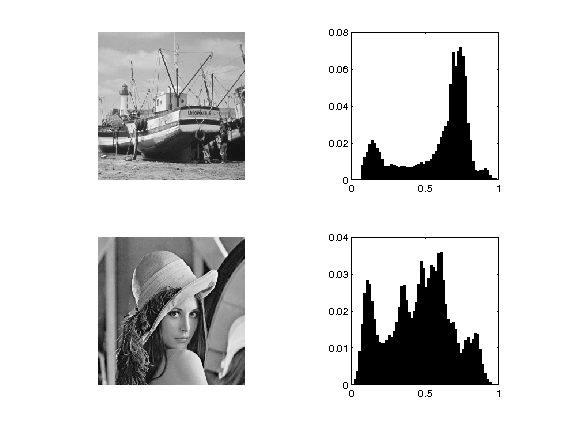Exercise 1

Histogram equalization is an orthogonal projector that maps the values of one signal onto the values of the other signal. This is achieved by assiging the sorted of ont signal to the sorted values of the other signla. Implement this for the two images.

In :
exo1()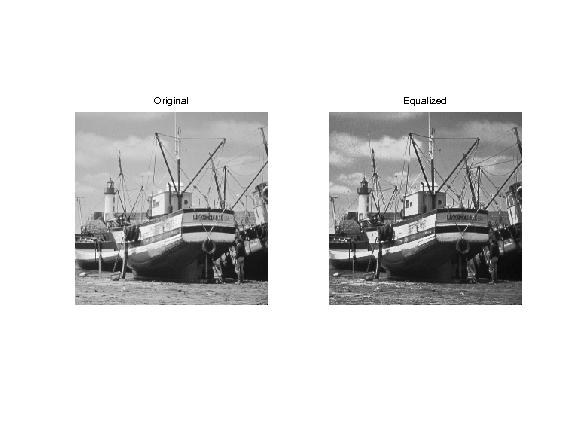In :
%% Insert your code here.


## Statistics of the Wavelets Coefficients of Natural Images¶

Although the histograms of images are flat, the histogram of their wavelet coefficients are usually highly picked at zero, resulting in a low entropy.

In :
n = 256*2;
M = rescale( load_image('lena', n) );


Compute its wavelet coefficients.

In :
Jmin = 4;
MW = perform_wavelet_transf(M,Jmin, +1);


Extract the fine horizontal details and display histograms. Take care at computing a centered histogram.

extract the vertical details

In :
MW1 = MW(1:n/2,n/2+1:n);


compute histogram

In :
v = max(abs(MW1(:)));
k = 20;
t = linspace(-v,v,2*k+1);
h = hist(MW1(:), t);


display

In :
clf;
subplot(1,2,1);
imageplot(MW1);
subplot(1,2,2);
bar(t, h/sum(h)); axis('tight');
axis('square');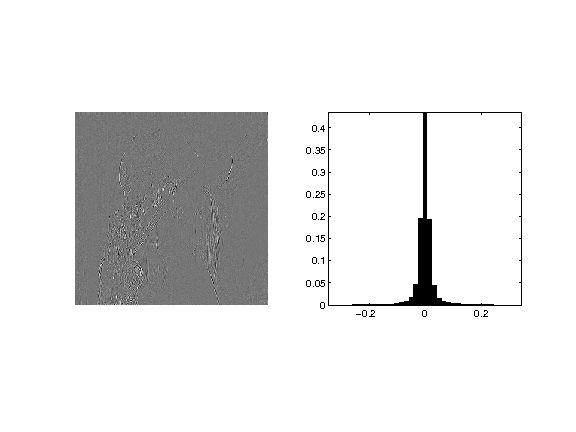## Higher Order Statistics¶

In order to analyse higher order statistics, one needs to consider couples of wavelet coefficients. For instance, we can consider the joint distribution of a coefficient and of one of its neighbors. The interesting quantities are the joint histogram and the conditional histogram (normalized so that row sum to 1).

Compute quantized wavelet coefficients.

In :
T = .03;
MW1q = floor(abs(MW1/T)).*sign(MW1);
nj = size(MW1,1);


Compute the neighbors coefficients.

spacial shift

In :
t = 2; % you can try with other values
C = reshape(MW1q([ones(1,t) 1:nj-t],:),size(MW1));


Compute the conditional histogram.

In :
options.normalize = 1;
[H,x,xc] = compute_conditional_histogram(MW1q,C, options);


Display.

In :
q = 8; % width for display
H = H((end+1)/2-q:(end+1)/2+q,(end+1)/2-q:(end+1)/2+q);
clf;
imagesc(-q:q,-q:q,max(log(H), -5)); axis image;
colormap gray(256);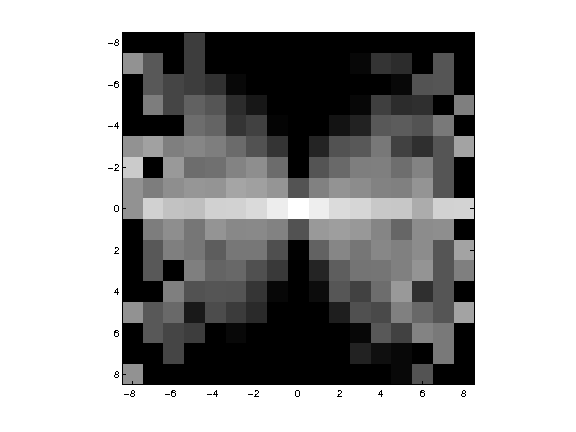Compute and display joint distribution.

In :
options.normalize = 0;
[H,x,xc] = compute_conditional_histogram(MW1q,C, options);
H = H((end+1)/2-q:(end+1)/2+q,(end+1)/2-q:(end+1)/2+q);


display level sets

In :
clf;
contour(-q:q,-q:q,max(log(H), -5), 20, 'k'); axis image;
colormap gray(256);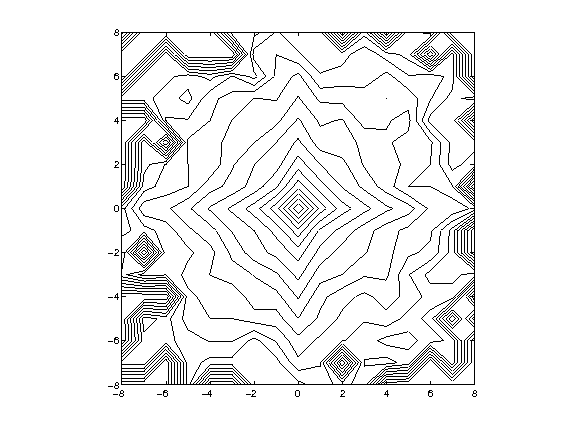## Conditional coding¶

Since the neighboring coefficients are typically un-correlated but dependant, one can use this dependancy to build a conditional coder. In essence, it amouts to using several coder, and coding a coefficient with the coder that corresponds to the neighbooring value. Here we consider 3 coder (depending on the sign of the neighbor).

Compute the contexts of the coder, this is the sign of the neighbor.

In :
C = sign( reshape(MW1q([1 1:nj-1],:),size(MW1)) );


Compute the conditional histogram

In :
[H,x,xc] = compute_conditional_histogram(MW1q,C);


Display the curve of the histogram

In :
clf; plot(x,H, '.-');
axis([-10 10 0 max(H(:))]);
legend('sign=-1', 'sign=0', 'sign=+1');
set_graphic_sizes([], 20);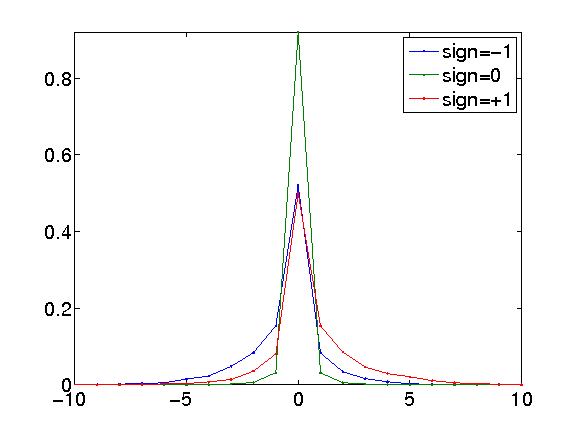Compare the entropy with/without coder.

global entropy (without context)

In :
ent_total = compute_entropy(MW1q);


compute the weighted entropy of this context coder

In :
h0 = compute_histogram(C);
H(H==0) = 1e-9; % avoid numerical problems
ent_partial = sum( -log2(H).*H );
ent_cond = sum( ent_partial.*h0' );


display the result

In :
disp(['Global coding: ' num2str(ent_total,3), ' bpp.']);
disp(['Conditional coding: ' num2str(ent_cond,3), ' bpp.']);

Global coding: 0.938 bpp.
Conditional coding: 0.829 bpp.